1. /
2. CBSE
3. /
4. Class 07
5. /
6. Mathematics
7. /
8. NCERT Solutions for Class...

# NCERT Solutions for Class 7 Maths Exercise 12.4### myCBSEguide App

Download the app to get CBSE Sample Papers 2023-24, NCERT Solutions (Revised), Most Important Questions, Previous Year Question Bank, Mock Tests, and Detailed Notes.

NCERT solutions for Maths Algebraic Expressions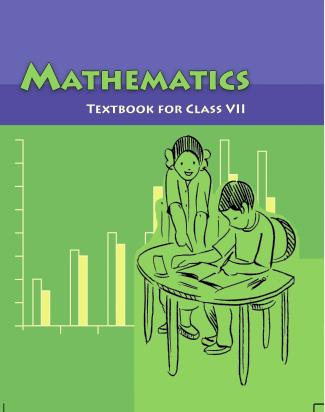## NCERT Solutions for Class 7 Maths Algebraic Expressions

###### Question 1.Observe the patterns of digits made from line segments of equal length. You will find such segmented digits on the display of electronic watches or calculators.

(a)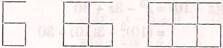… …

1. 11 16 21… {tex}\left( {5n + 1} \right)…{/tex}

(b)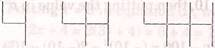1. 7 10 13… {tex}\left( {3n + 1} \right)…{/tex}

(c)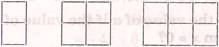7 121722…

If the number of digits formed is taken to be {tex}n,{/tex} the number of segments required to form {tex}n{/tex} digits is given by the algebraic expression appearing on the right of each pattern.

How many segments are required to form 5, 10, 100 digits of the kind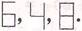S. No. Symbol Digit’s number Pattern’s Formulae No. of Segments (i)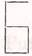5 {tex}5n + 1{/tex} 26 10 51 100 501 (ii)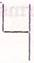5 {tex}3n + 1{/tex} 16 10 31 100 301 (iii)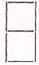5 {tex}5n + 2{/tex} 27 10 52 100 502

Putting {tex}n = 5,{/tex} 5 x 5 + 1 = 25 + 1 = 26(i) {tex}5n + 1{/tex}

Putting {tex}n = 10,{/tex} 5 x 10 + 1 = 50 + 1 = 51

Putting {tex}n = 100,{/tex} 5 x 100 + 1 = 500 + 1 = 501

(ii) {tex}3n + 1{/tex}

Putting {tex}n = 5,{/tex} 3 x 5 + 1 = 15 + 1 = 16

Putting {tex}n = 10,{/tex} 3 x 10 + 1 = 30 + 1 = 31

Putting {tex}n = 100,{/tex} 3 x 100 + 1 = 300 + 1 = 301

(iii) {tex}5n + 2{/tex}

Putting {tex}n = 5,{/tex} 5 x 5 + 2 = 25 + 2 = 27

Putting {tex}n = 10,{/tex} 5 x 10 + 2 = 50 + 2 = 52

Putting {tex}n = 100,{/tex} 5 x 100 + 2 = 500 + 2 = 502

NCERT Solutions for Class 7 Maths Exercise 12.4

###### Question 2.Use the given algebraic expression to complete the table of number patterns:
 S.No. Expression Terms 1st 2nd 3rd 4th 5th … 10th … 100th … (i) {tex}2n – 1{/tex} 1 3 5 7 9 — 19 — — — (ii) {tex}3n + 2{/tex} 2 5 8 11 — — — — — — (iii) {tex}4n + 1{/tex} 5 9 13 17 — — — — — — (iv) {tex}7n + 20{/tex} 27 34 41 48 — — — — — — (v) {tex}{n^2} + 1{/tex} 2 5 10 17 — — — — 10001 —

Putting {tex}n = 100,{/tex} 2 x 100 – 1 = 200 – 1 = 199

(ii) {tex}3n + 2{/tex}

Putting {tex}n = 5,{/tex} 3 x 5 + 2 = 15 + 2 = 17

Putting {tex}n = 10,{/tex} 3 x 10 + 2 = 30 + 2 = 32

Putting {tex}n = 100,{/tex} 3 x 100 + 2 = 300 + 2 = 302

(iii) {tex}4n + 1{/tex}

Putting {tex}n = 5,{/tex} 4 x 5 + 1 = 20 + 1 = 21

Putting {tex}n = 10,{/tex} 4 x 10 + 1 = 40 + 1 = 41

Putting {tex}n = 100,{/tex} 4 x 100 + 1 = 400 + 1 = 401

(iv) {tex}7n + 20{/tex}

Putting {tex}n = 5,{/tex} 7 x 5 + 20 = 25 + 20 = 55

Putting {tex}n = 10,{/tex} 7 x 10 + 20 = 70 + 20 = 90

Putting {tex}n = 100,{/tex} 7 x 100 + 20 = 700 + 20 = 720

(v) {tex}{n^2} + 1{/tex}

Putting {tex}n = 5,{/tex} 5 x 5 + 1 = 25 + 1 = 26

Putting {tex}n = 10,{/tex} 10 x 10 + 1 = 100 + 1 = 101

Putting {tex}n = 100,{/tex} 100 x 100 + 1 = 10000 + 1 = 10001

Now complete table is,

 S.No. Expression Terms 1st 2nd 3rd 4th 5th … 10th … 100th … (i) {tex}2n – 1{/tex} 1 3 5 7 9 — 19 — 199 — (ii) {tex}3n + 2{/tex} 2 5 8 11 17 — 32 — 302 — (iii) {tex}4n + 1{/tex} 5 9 13 17 21 — 41 — 401 — (iv) {tex}7n + 20{/tex} 27 34 41 48 55 — 90 — 720 — (v) {tex}{n^2} + 1{/tex} 2 5 10 17 26 — 101 — 10001 —

## NCERT Solutions for Class 7 Maths Exercise 12.4

NCERT Solutions for Class 7 Maths Exercise 12.4 PDF (Download) Free from myCBSEguide app and myCBSEguide website. Ncert solution class 7 Maths includes text book solutions from Class 7 Maths Book . NCERT Solutions for CBSE Class 7 Maths have total 15 chapters. 7 Maths NCERT Solutions in PDF for free Download on our website. Ncert Maths class 7 solutions PDF and Maths ncert class 7 PDF solutions with latest modifications and as per the latest CBSE syllabus are only available in myCBSEguide.

## CBSE app for Students

To download NCERT Solutions for Class 7 Maths, Social Science Computer Science, Home Science, Hindi English, Maths Science do check myCBSEguide app or website. myCBSEguide provides sample papers with solution, test papers for chapter-wise practice, NCERT solutions, NCERT Exemplar solutions, quick revision notes for ready reference, CBSE guess papers and CBSE important question papers. Sample Paper all are made available through the best app for CBSE students and myCBSEguide website.### Test Generator

Create question paper PDF and online tests with your own name & logo in minutes.### myCBSEguide

Question Bank, Mock Tests, Exam Papers, NCERT Solutions, Sample Papers, Notes